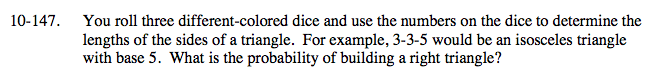### Home > A2C > Chapter 10 > Lesson 10.2.6 > Problem10-147

10-147.

You roll three different-colored dice and use the numbers on the dice to determine the lengths of the sides of a triangle. For example, 3-3-5 would be an isosceles triangle with base 5. What is the probability of building a right triangle? Homework Help ✎What right triangles have integral side values less than or equal to 6?

3, 4, 5

How many different orders result in the right triangle?

6

Now how many different combinations are there total.

216

$\frac{1}{36}$